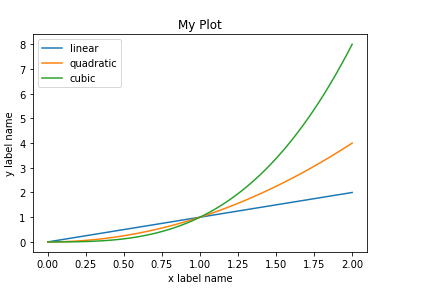# How can matplotlib be used to plot 3 different datasets on a single graph in Python?

Matplotlib is a popular Python package that is used for data visualization. Visualizing data is a key step since it helps understand what is going on in the data without actually looking at the numbers and performing complicated computations. It helps in communicating the quantitative insights to the audience effectively.

Matplotlib is used to create 2 dimensional plots with the data. It comes with an object−oriented API that helps in embedding the plots in Python applications. Matplotlib can be used with IPython shells, Jupyter notebook, Spyder IDE and so on. It is written in Python. It is created using Numpy, which is the Numerical Python package in Python.

Python can be installed on Windows using the below command −

pip install matplotlib

The dependencies of Matplotlib are −

Python ( greater than or equal to version 3.4)
NumPy
Setuptools
Pyparsing
Libpng
Pytz
Free type
Six
Cycler
Dateutil

Let us understand how Matplotlib can be used to plot 3 different data sets in a single plot −

## Example

import matplotlib.pyplot as plt
import numpy as np
fig = plt.figure()
x = np.linspace(0, 2, 100)
fig, ax = plt.subplots()
ax.plot(x, x, label='linear')
ax.plot(x, x**3, label='cubic')
ax.set_xlabel('x label name')
ax.set_ylabel('y label name')
ax.set_title("My Plot")
ax.legend()

## Output## Explanation

• The required packages are imported and its alias is defined for ease of use.

• An empty figure is created using the ‘figure’ function.

• The data is created using NumPy package.

• The ‘subplot’ function is used to create outlines for three different plots.

• The type of plot for each of the three datasets is defined.

• The labels for ‘X’ and ‘Y’ axis is defined.

• The title of the plot is defined, and it is shown on the console.